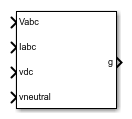# PWM Generator (Vienna Rectifier)

Vienna rectifier pulse-width modulation waveform generator

Since R2019b

•Libraries:
Simscape / Electrical / Control / Pulse Width Modulation

## Description

The PWM Generator (Vienna Rectifier) block:

1. Calculates on-gating and off-gating times based on the block inputs:

• Three sinusoidal reference voltages, one per phase, that must be generated at the AC side of the Vienna rectifier, one per phase

• Three filtered input sinusoidal current measurements of the Vienna rectifier, one per phase

• A DC-link neutral point balance control signal

2. Uses the gating times to generate three switch-controlling pulses.

The block uses a simplified space vector modulation method based on the equivalence between two-level and three-level converters to generate the control pulses .

The three-phase filtered input currents are used to identify the active voltage vector hexagons. The switching sequence selection and on-gating and off-gating times are determined by simplifying the space-vector diagram of a three-level inverter into that of a two-level inverter .

The DC link neutral point balance control signal that comes from the external DC link neutral control loop is used to adjust the time distribution of the redundant voltage vectors within one single switching period.

### Sampling Mode

The block allows you to choose natural, symmetric, or asymmetric sampling of the modulation wave.

The PWM Generator (Vienna Rectifier) block does not perform carrier-based pulse-width modulation (PWM). Instead, the block uses input signals to calculate gating times and uses the gating times to generate the switch-controlling pulses.

## Ports

### Input

expand all

Three sinusoidal voltages, one per phase.

Three currents, one per phase.

DC link voltage of the converter. The value of the signal must be greater than 0.

Output of the feedback-control loop that balances the DC supply. The value of the signal must be between `–1` and `+1`.

### Output

expand all

Three-pulse waveforms that determine the switching behavior in the attached power converter.

## Parameters

expand all

Sampling mode that determines whether the block samples the modulation waveform when the waves intersect or when the carrier wave is at one or both of its boundary conditions.

Rate at which the switches in the power converter switch, in Hz.

Time interval between successive block executions, in s. To ensure adequate resolution in the generated signal, set this value to be less than or equal to `1/(50*Fsw)`, where Fsw is the Switching frequency (Hz).

 R. Burgos, R. Lai, Y. Pei, F.Wang, D. Boroyevich, and J. Pou, “Space vector modulation for Vienna-type rectifiers based on the equivalence between two- and three-level converters: A carrier-based implementation”, IEEE Trans. Power Electron., vol. 23, no. 4, pp. 1888–1898, Jul. 2008

 J. H. Seo, C. H. Choi, and D. S. Hyun, “A new simplified space-vector PWM method for three-level inverters”, IEEE Trans. Power Electron., vol. 16, no. 4, pp. 545–550, Jul. 2001.